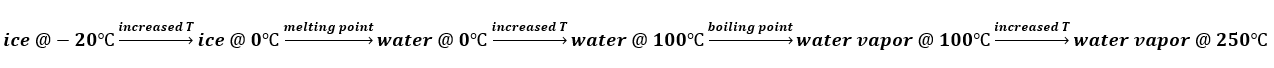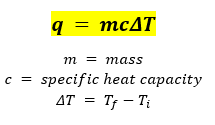# Problem: What quantity of energy does it take to convert 0.500 kg ice at -20. ˚C to steam at 250. ˚C? Specific heat capacities: ice, 2.03 J/g • ˚C; liquid, 4.2 J/g • ˚C; steam, 2.0 J/g • ˚C; ΔHvap = 40.7 kJ/mol; ΔHfus = 6.02 kJ/mol.

🤓 Based on our data, we think this question is relevant for Professor Kirby's class at Quinnipiac University.

###### FREE Expert Solution

The conversion of ice at -20°C to stem at 250°C happens in 5 steps as shown below:Calculate the heat required for each step:

Use the following equation for the increasing temperature step:Calculate first the amount of heat required to increase the temperature of ice from -20°C to 0°C:###### Problem Details

What quantity of energy does it take to convert 0.500 kg ice at -20. ˚C to steam at 250. ˚C? Specific heat capacities: ice, 2.03 J/g • ˚C; liquid, 4.2 J/g • ˚C; steam, 2.0 J/g • ˚C; ΔHvap = 40.7 kJ/mol; ΔHfus = 6.02 kJ/mol.